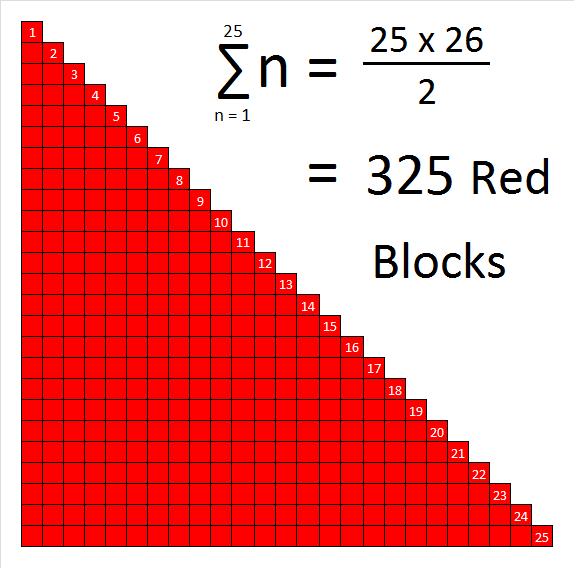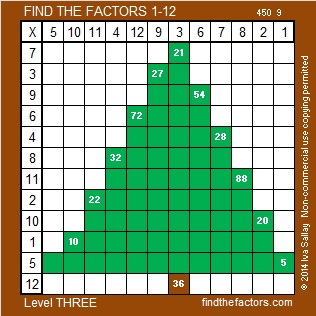# 325 is a Triangular Number

• 325 is a composite number.
• Prime factorization: 325 = 5 x 5 x 13, which can be written 325 = 5² x 13
• The exponents in the prime factorization are 2 and 1. Adding one to each and multiplying we get (2 + 1)(1 + 1) = 3 x 2  = 6. Therefore 325 has exactly 6 factors.
• Factors of 325: 1, 5, 13, 25, 65, 325
• Factor pairs: 325 = 1 x 325, 5 x 65, or 13 x 25
• Taking the factor pair with the largest square number factor, we get √325 = (√13)(√25) = 5√13 ≈ 18.028325 is a triangular number. 1 + 2 + 3 + 4 + . . . + 22 + 23 + 24 + 25 = 325. Shorthand for that sum of 25 numbers is given with the ∑ sign in the graphic below. The way to find the sum quickly using multiplication is also given:If you look at this Level 3 puzzle from far enough away, you also might see a triangle!Print the puzzles or type the factors on this excel file: 12 Factors 2014-12-15

A Logical Approach to FIND THE FACTORS: Find the column or row with two clues and find their common factor. Write the corresponding factors in the factor column (1st column) and factor row (top row).  Because this is a level three puzzle, you have now written a factor at the top of the factor column. Continue to work from the top of the factor column to the bottom, finding factors and filling in the factor column and the factor row one cell at a time as you go.## 3 thoughts on “325 is a Triangular Number”

1.Paula Beardell Krieg

So is 3 the first triangular number, and 6 the next one?

2.ivasallay

Almost. The first triangular number is 1.
3 is the second, and 6 is the third.
I’ve updated the graphic to make that more clear. 325 is the 25th triangular number.
The nth triangular number can be found by adding up the first n integers or by finding n(n+1)/2.

This site uses Akismet to reduce spam. Learn how your comment data is processed.